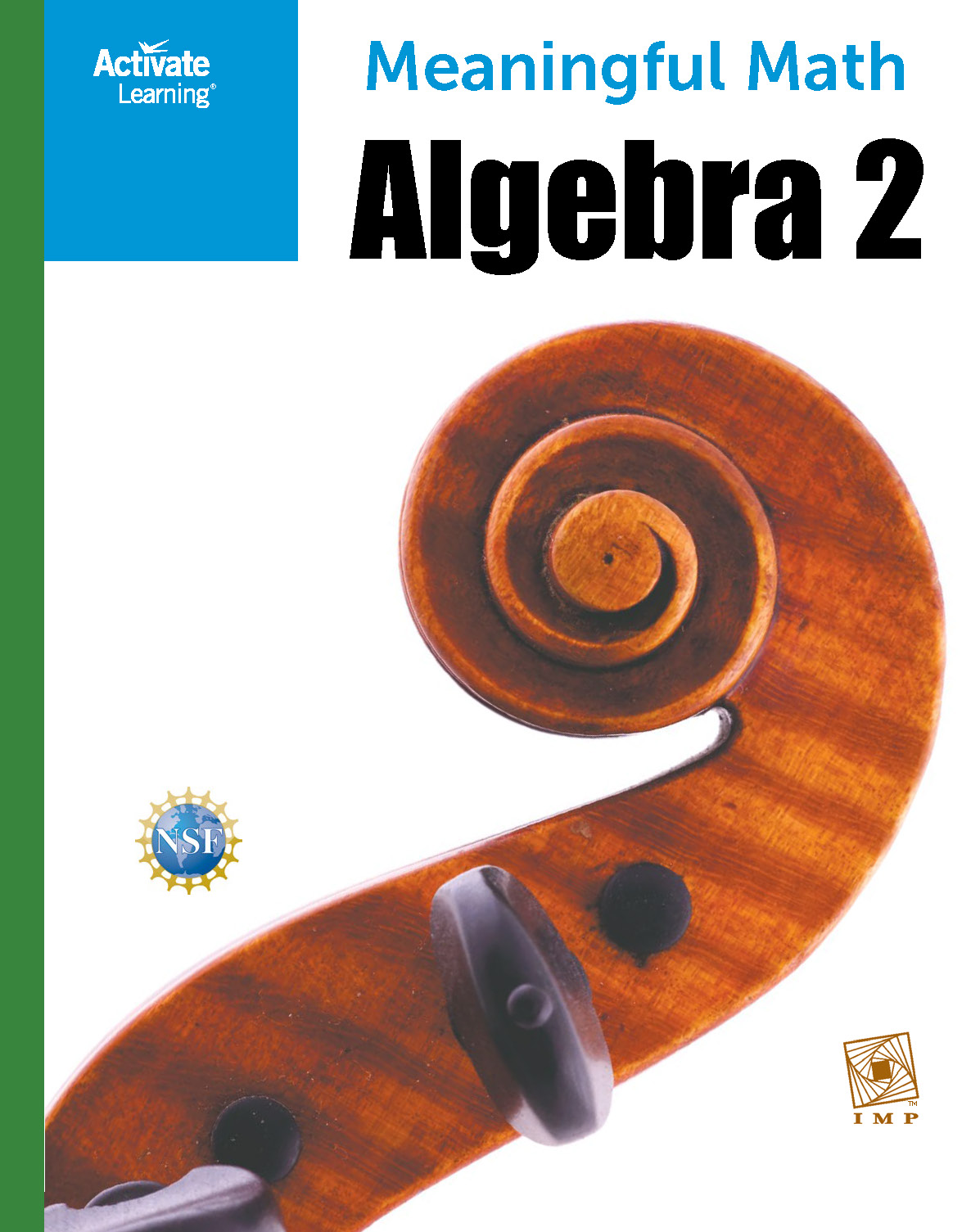# Algebra 2

SMALL WORLD, ISN'T IT? Beginning with a table of population data, students study situations involving rates of growth, develop the concept of slope, and then generalize this to the idea of the derivative.

THE GAME OF PIG Students develop a mathematical analysis for a complex game based on an area model for probability.

HIGH DIVE Using trigonometry, polar coordinates, and the physics of falling objects, students model this problem: When should a diver on a Ferris wheel aiming for a moving tub of water be released in order to create a splash instead of a splat?

THE WORLD OF FUNCTIONS In this unit, students explore families of functions in terms of various representations—tables, graphs, algebraic representations, and situations they can model; they also explore ways of combining functions using arithmetic operations and composition.

IS THERE REALLY A DIFFERENCE? Students build on prior experience with statistical ideas expanding their understanding of statistical analysis.

You must be logged in to use this feature. LOGIN

Phone: 646.502.5231 | EMail: 'support@activatelearning.com'